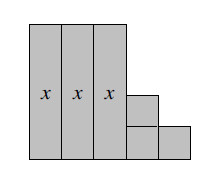### Home > MC2 > Chapter 4 > Lesson 4.2.1 > Problem4-32

4-32.

Sketch the algebra tile shape shown below on your paper. Write expressions for the area and perimeter of the shape. Then, calculate the area and perimeter of the shape for each $x$-value.

1. $x = 9$ cm

1. $x = 0.5$ cm

1. $x = 15$ cmAdd the areas of all $6$ tiles to find the shape's total area.

Add the length of all the sides of the shape to find the perimeter.

Try labeling all the sides first.

Area: $3x+3$

Perimeter: $2x + 10$

$x=9$ cm
Substitute $9$ cm for $x$ in both equations.

A $= 3 (9) + 3$
P $= 2 (9) + 10$
Use order of operations to simplify and find the answer.

A $= 30$ sq cm
P $= 28$ cm

$x = 0.5$ cm
See part (a).

A $= 4.5$ sq cm
P $= 11$ cm

See part (a).